# How to Add Margining Capability to a DC-DC Converter

### Abstract

This application note explains how easy it is to add margining capability to a DC-DC converter by connecting it to a DS4404 4-channel adjustable current DAC (or the DS4402, 2-channel version).

This application note shows how to connect the DS4404, a 4-channel adjustable current DAC (or the DS4402, a 2-channel version of the DS4404), to a DC-DC converter circuit to add margining capability.

The circuit in Figure 1 illustrates how simple it is to integrate the DS4404 into an existing design. The DS4404 is added to the feedback node (see the dashed line) so that the VOUT of the DC-DC converter can be adjusted. On power up, the DS4404 outputs a current of 0A (appears as high impedance), essentially making the DS4404 transparent until it is written to through I²C.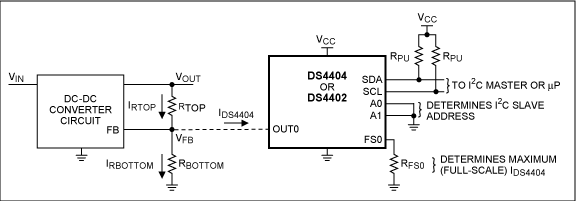Figure 1. Connecting a DS4404 to a DC-DC converter feedback circuit.

Assume the following for our example (which are independent of the DS4404):

VIN = 3V to 5.5V
VOUT = 1.8V (desired nominal output voltage)
VFB = 0.6V (not to be confused with VREF of the DS4404)

The value of VFB is found in the DC-DC converter's data sheet. It is important to verify that this voltage is within the OUTx voltage range specified in the DS4404's data sheet (VOUT:SINK and VOUT:SOURCE). Finally, it is also important to check the input impedance of the DC-DC converter's FB pin; the example here assumes that it is high impedance.

Assume that we are using the DS4404 to add ±20% margining of VOUT. Therefore, we have:

VOUTMAX = 2.16V
VOUTNOM = 1.8V
VOUTMIN = 1.44V

Begin by determining the necessary relationship between RTOP and RBOTTOM which yield nominal VOUT, VOUTNOM, when IDS4404 = 0A.Solving for RTOP, we get: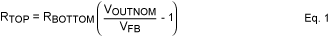So for our example: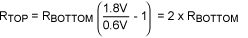The current required to make VOUT increase to VOUTMAX, IDS4404, is derived by summing the currents at the FB node.This equation can be simplified by solving Equation 1 for RBOTTOM and then substituting.Or in terms of margin percentage: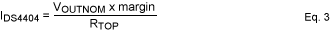where margin = 0.2 for this example of ±20% margining.

However, before we can use this relationship to calculate RTOP and RBOTTOM, the full-scale current, IFS, must be selected.

According to the DS4404's data sheet, the full-scale current needs to be between 0.5mA and 2.0mA (specified as IOUT:SINK and IOUT:SOURCE in the DS4404 data sheet, depending on whether sinking or sourcing current) to assure the accuracy and linearity specifications. Unfortunately, there is no one formula to calculate the ideal full-scale current. Every application will be different. Some items that influence the selection of the full-scale current are the desired number of steps, step size, as well as RTOP and RBOTTOM values. Likewise, there may be times when you want a particular register setting to correspond to a particular margin percentage. Either way, selecting the ideal full-scale current for your application will usually take several iterations of arbitrarily selecting a full-scale current (within the range) and then calculating RTOP, RBOTTOM, RFS, and step size. Then, once a full-scale current is determined, you can choose to adjust it or some of the resistor values in order to end up with common resistor values.

Returning to the original example, to calculate RTOP we will make IFS = IDS4404. This will give us 31 steps from VOUTNOM to VOUTMAX and 31 steps from VOUTNOM to VOUTMIN, which is more than adequate for our example.

Alternatively, we could begin by arbitrarily choosing IFS to be in the center (1.25mA) of the specified range, and then perform all the calculations. Instead, for illustrative purposes we will perform the calculations for the endpoints of the range.

So for IFS = IDS4404 = 0.5mA.

Using Eq. 3 and solving for RTOP: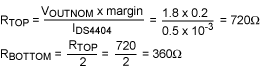Then RFS is calculated using the formula given in the DS4404 data sheet along with VREF, also found in the DS4404 data sheet: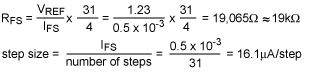Finally, for completeness we can determine the DS4404 output current as a function of register setting:Note: the register setting here does not include the sign bit, which is used to select sink or source. The DS4404 sinks current when the sign bit = 0, thus making VOUT increase to VOUTMAX. The DS4404 sources current when the sign bit = 1, thus decreasing VOUT towards VOUTMIN.

Likewise for IFS = IDS4404 = 2.0mA: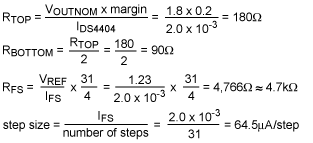Comparing RTOP and RBOTTOM of the two cases, one can see that IFS = 0.5mA is more attractive because the resistances are higher.

A similar article was published September 18, 2008 on the EDN website.